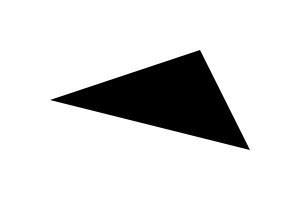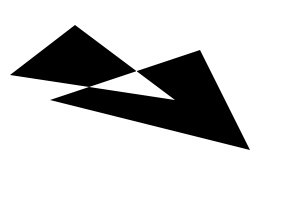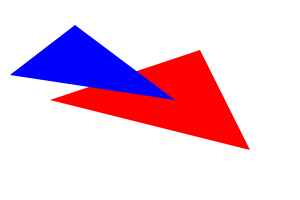# 3. Tutorial

(This section is unfinished.)

The cl-aa-misc package provides some functions for creating images and performing very basic operation on them (loading and saving them.) It is not intended to be used outside of this tutorial. Images can only be 24bits RGB (with 8 bits integer per component.)

The show-image function used in this tutorial expect a path to an external program to be specified in aa-misc:*external-viewer*. This program will be used to display PNM pictures. Under Unix, xv may be a good choice, and it is the default.

Assuming you're using an implementation which use ASDF with require, you must load cl-aa-misc and cl-vectors as follow:

Systems required for this tutorial
```(require 'asdf)
(require 'cl-aa-misc)
(require 'cl-vectors)
```

This first bit of code create a blank image and display it.

create and display a blank image
```(let ((image (aa-misc:make-image 300 200 #(255 255 255))))
(aa-misc:show-image image))
```

This creates a 300x200 images with the white color. The color is specified with a 3-elements array with values between 0 and 255. Note: Actually the image is just an Common Lisp array of dimensions (300 200 3) with (unsigned-byte 8) elements.

To save the image use (aa-misc:save-image filename image format) where format must be :pnm since nothing else is supported.

Given that, if we have to draw several pixels of the same color but with various opacity, we can build a function closure with the aa-misc:image-put-pixel function. Such a function will be necessary to render antialiased polygons.

The following example draw 4 pixels with various opacity.

draw some pixels with various opacity
```;;; Note that the image size is 4x3. Zoom it when it is displayed.
(let* ((image (aa-misc:make-image 4 3 #(255 255 255)))
(put-pixel (aa-misc:image-put-pixel image #(0 0 0))))
(funcall put-pixel 2 1 256)  ; full opacity
(funcall put-pixel 1 2 128)  ; half opacity
(funcall put-pixel 3 2 192)  ; 3/4 opacity
(funcall put-pixel 0 1 0)    ; null opacity, nothing drawn
(aa-misc:show-image image))
```

Now, something more interesting, we want to draw an antialiased polygon.

At the lowest level of this library, to draw some polygons we must create an "AA" state for the rasterizer, describe the polygon to draw, then draw the result. This can be done as follow:

draw a black triangle with antialiasing
```(let ((state (aa:make-state)))       ; create the state
(aa:line-f state 200 50 250 150)   ; describe the 3 sides
(aa:line-f state 250 150 50 100)   ; of the triangle
(aa:line-f state 50 100 200 50)
(let* ((image (aa-misc:make-image 300 200 #(255 255 255)))
(put-pixel (aa-misc:image-put-pixel image #(0 0 0))))
(aa:cells-sweep state put-pixel) ; render it
(aa-misc:show-image image)))
```

which produces:The algorithm computed the coverage of all the pixels involved to describe the triangle. The pixels inside the triangle are covered completly, and thus call our put-pixel function with 256 for the alpha value. For the pixels outside, the alpha is 0. For the pixels along the borders of the triangle it is between these 2 values, hence providing antialiasing information.

Note that the triangle was drawn clockwise.

If we now draw another triangle overlapping the first but counter-clockwise, this create a hole, because the algorithm which compute pixel coverage will have cancelled the overlapping zone. The alpha value will be 0 at the intersection because the first triangle produces alpha of 256 while the counter-clockwise triangle produces alpha of -256. FIXME: Find a better explanation.

2 overlapping triangles
```(let ((state (aa:make-state)))       ; create the state
;; the 1st triangle
(aa:line-f state 200 50 250 150)   ; describe the 3 sides
(aa:line-f state 250 150 50 100)   ; of the first triangle
(aa:line-f state 50 100 200 50)
;; the 2nd triangle
(aa:line-f state 75 25 10 75)      ; describe the 3 sides
(aa:line-f state 10 75 175 100)    ; of the second triangle
(aa:line-f state 175 100 75 25)
(let* ((image (aa-misc:make-image 300 200 #(255 255 255)))
(put-pixel (aa-misc:image-put-pixel image #(0 0 0))))
(aa:cells-sweep state put-pixel) ; render it
(aa-misc:show-image image)))
```

which produces:The function put-pixel is only called for the pixels on the border of the various polygons. It is also called for the interior of the polygons, but we can provide an optimized version of the function in this case which can handle a run of pixels of the same opacity. See the section "cl-aa" for more information about that.

Note that we could not have drawn the triangles with different color (one red and one blue for example.) Since it is happenning in a single state, everything is rendered with a specific filling method. As we will see later in this tutorial (yet to be written..), this library provides some way to fill polygon with gradient (radial or linear) and with textures (either from picture or from previously rendered polygons.)

To draw 2 triangles with different colors, we must render them like this:

red and blue triangles
```(let ((state1 (aa:make-state))
(state2 (aa:make-state)))
;; the 1st triangle
(aa:line-f state1 200 50 250 150)   ; describe the 3 sides
(aa:line-f state1 250 150 50 100)   ; of the first triangle
(aa:line-f state1 50 100 200 50)
;; the 2nd triangle
(aa:line-f state2 75 25 10 75)      ; describe the 3 sides
(aa:line-f state2 10 75 175 100)    ; of the second triangle
(aa:line-f state2 175 100 75 25)
(let ((image (aa-misc:make-image 300 200 #(255 255 255))))
(aa:cells-sweep state1 (aa-misc:image-put-pixel image #(255 0 0)))
(aa:cells-sweep state2 (aa-misc:image-put-pixel image #(0 0 255)))
(aa-misc:show-image image)))
```

which produces:But by using some special way to handle the alpha value (with a specific put-pixel function), we could obtains the colored version with the hole at the same time by testing the sign of the alpha value (which is normally considered only for its absolute value) to decide to choose between the 2 colors. And this is not the only way to achieve that.

Generated by CL-Crock on 2010-09-25T15:20:58Z